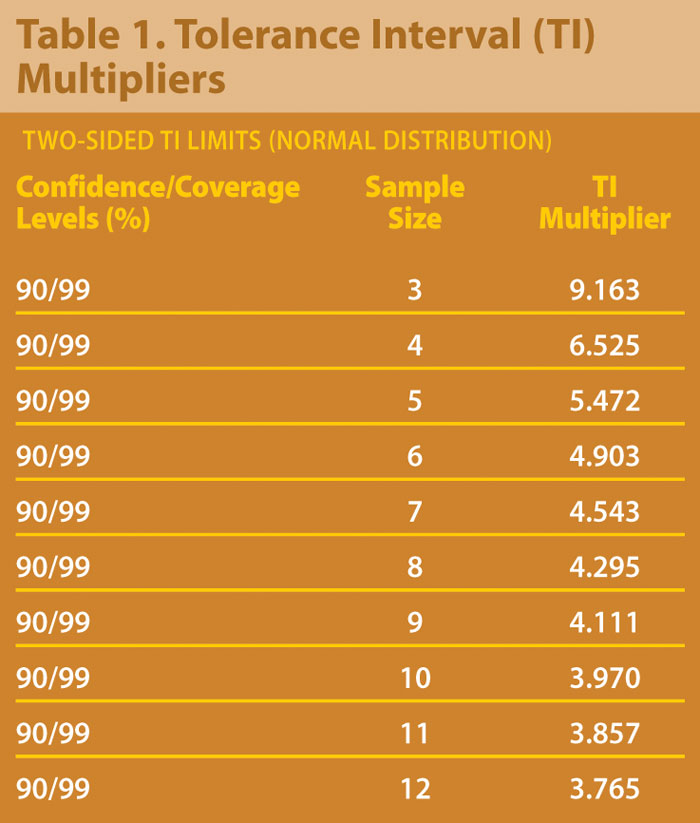November 1, 2010 (Vol. 30, No. 19)

Keith M. Bower
Abe Germansderfer

Statistical Strategies to Address the Challenges Imposed by Limited Data

To establish whether clinical-product quality remains constant when making a process change can be a challenging exercise. Limited data availability further complicates the assessment of whether the two populations (pre-change and post-change) are comparable.

As discussed in ICH Guidance Q5E, it is not a requirement to show that quality attributes of the pre- and post-change populations are identical, rather that “they are highly similar and that the existing knowledge is sufficiently predictive to ensure that any differences in quality attributes have no impact upon safety or efficacy of the drug product.”

Historically, comparability is determined using a variety of statistical techniques. These may include, but are not limited to: student’s independent two-sample t-test, statistical equivalency tests, and statistical tolerance intervals.

This article addresses the principle behind each approach. Further, we consider a risk-based approach to determine an appropriate strategy for setting comparability criteria, based on probability theory.Statistical Approaches

Student’s independent two-sample t-test

The appropriation of the student’s t-test for demonstrating comparability involves two distributions that are assumed to be approximately well modeled by normal distributions (note that the procedure may be relatively robust to the assumption of Normality). The null hypothesis for the test assumes equality across the means, with the alternative hypothesis typically assuming unequal means. The p-value calculated from the test statistic is compared with the prescribed significance level (typically 5%). If the p-value exceeds the significance level, comparability is assumed.

Importantly, it is recognized that this approach of “proving quality” by failing to reject a null hypothesis is flawed. Under hypothesis testing, the “burden of proof” falls on the null hypothesis. Such tests are only able to reject the null hypothesis.  Based on this description, the null hypothesis counter-intuitively sets up the test to reject the comparability the experimenter aims to demonstrate.

Most practitioners recognize that when it comes to statistical significance, the absence of evidence to reject the null hypothesis and declare inequality is not evidence of equality. In other words, p-values greater than the prescribed significance level are not demonstrative of comparability.

Statistical equivalency tests

Statistical equivalency tests, e.g., two one-sided t-tests (TOST) are widely accepted as the preferred method for demonstrating comparability. In contrast with the student’s independent two-sample t-test approach, the null and alternative hypotheses are correctly designed to test for equivalency.

In particular, the null hypothesis using TOST is such that the difference between the two parameters (e.g., two population means) exceeds a comparability criteria, typically called the goalpost (θ). The two null hypotheses in TOST can be written as:

H01: µ1 – µ2 ≤ θ, and H02: µ1– µ2 ≥ θ where µ1 represents the pre-change mean, and µ2 represents the post-change mean.

Statistical equivalency is demonstrated if the two one-sided upper 95% confidence limits for the difference between the two means both fall inside the equivalency region (-θ, θ,).

The amount of data collected should ensure the TOST procedure is adequately powered. However, when only limited data is available (e.g., when the process yields are great enough during early development such that only a small number of lots are required to supply clinical trials) TOST may be unable to declare equivalency even when population means are identical.sta

Statistical tolerance intervals

A statistical tolerance interval (TI) may be calculated using the pre-change data to set the comparability criteria. A TI covers a proportion (p) of a distribution, e.g., a normal distribution, for a given confidence level. For example, a 95/99% TI covers the middle 99% of a population with 95% confidence. To establish comparability using this approach, data from the post-change process is required to be completely contained inside the TI.

TI calculations take a similar form as those for confidence intervals for the mean. The formula may be written x + ks where x represents the sample mean from the pre-change process, s represents the sample standard deviation, and k is the tolerance interval multiplier.

Table 1 shows multipliers for 90% confidence for 99% of a normal population calculated using SAS 9.1.3.

Despite the ease of calculating comparability criteria using this approach, note that the TI approach has several disadvantages in comparison with TOST. These include:

• TI approach is not a mathematically derived hypothesis-based test. No p-value is generated to test hypotheses.
• Practitioners are “rewarded” when calculating a TI using smaller amounts of data, i.e., the TI multiplier is larger, making it easier to pass comparability.
• Comparability is more difficult to correctly show with increasing “new process” data (as one or more values could fall outside the interval by chance alone).Table 1. Tolerance interval (TI) multipliersThis site uses Akismet to reduce spam. Learn how your comment data is processed.